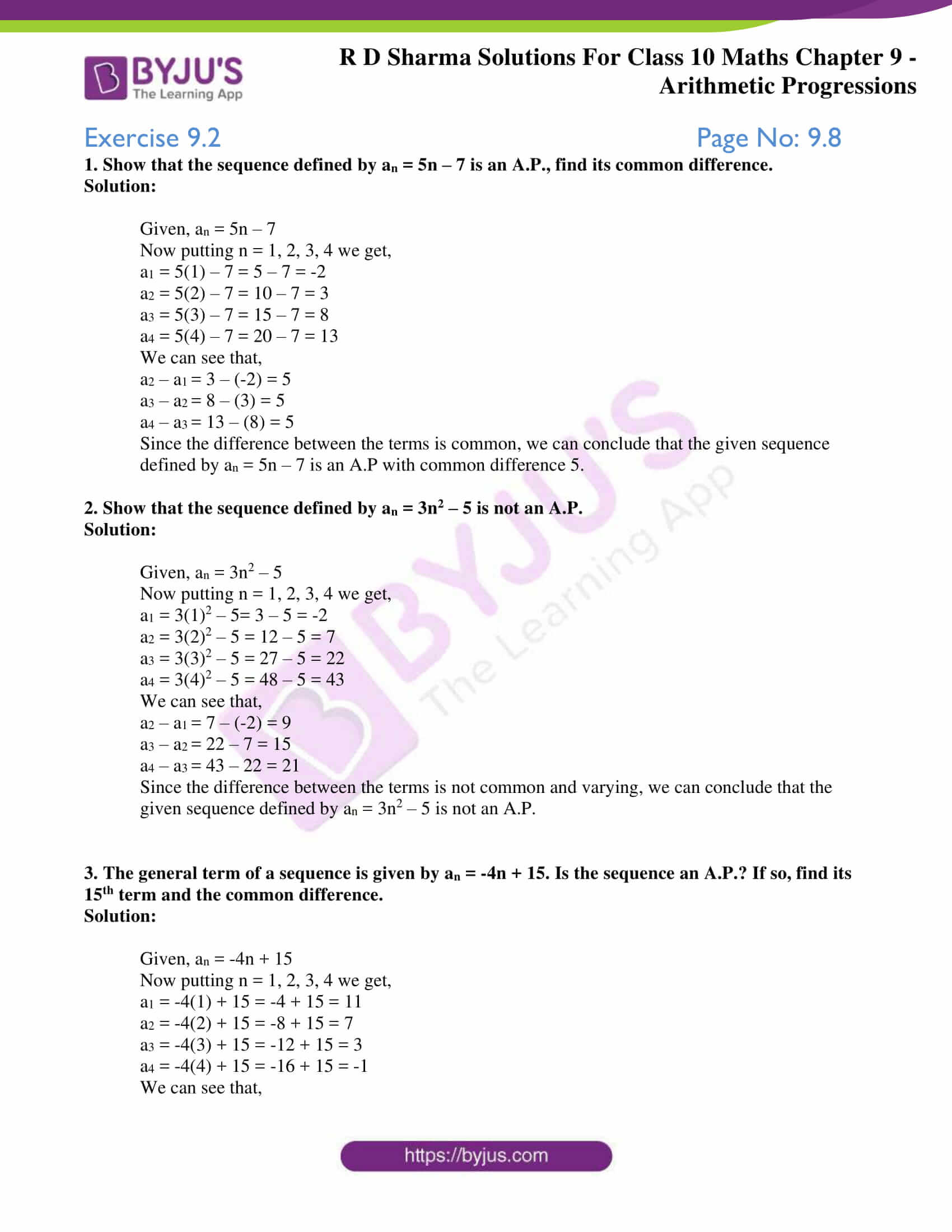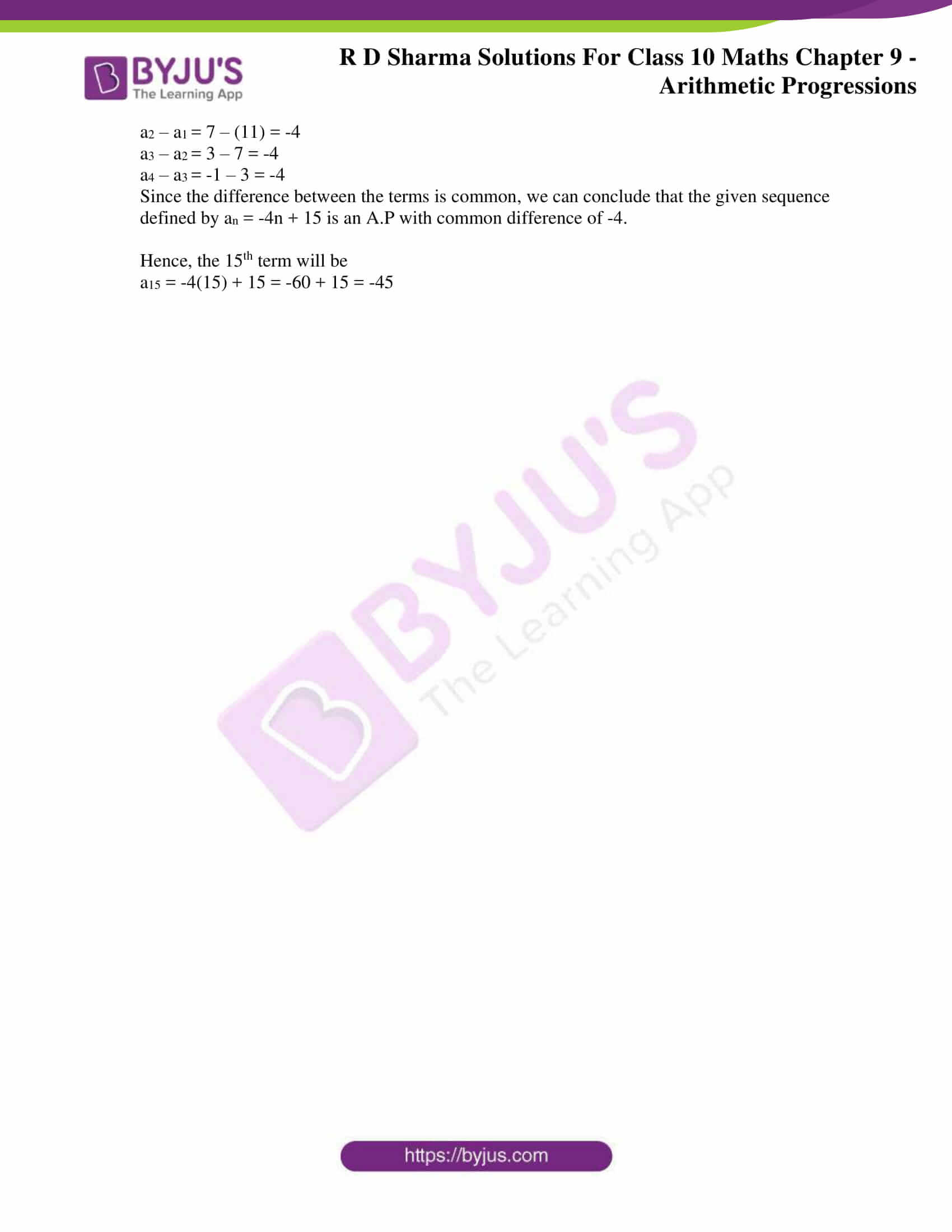# RD Sharma Solutions for Class 10 Maths Chapter 9 Arithmetic Progressions Exercise 9.2

The second exercise of the chapter is based on understanding the definition and properties of an A.P. The fundamentals of A.P will be strengthened when a student refers to RD Sharma Solutions Class 10 as it contains detailed solutions to problems aiming to clear doubts. Students can also access the RD Sharma Solutions for Class 10 Maths Chapter 9 Arithmetic Progressions Exercise 9.2 PDF provided below for further clarity in solving problems of this exercise.

## RD Sharma Solutions for Class 10 Maths Chapter 9 Arithmetic Progressions Exercise 9.2 Download PDF### Access RD Sharma Solutions for Class 10 Maths Chapter 9 Arithmetic Progressions Exercise 9.2

1. Show that the sequence defined by an = 5n – 7 is an A.P., find its common difference.

Solution:

Given, an = 5n – 7

Now putting n = 1, 2, 3, 4 we get,

a1 = 5(1) – 7 = 5 – 7 = -2

a2 = 5(2) – 7 = 10 – 7 = 3

a3 = 5(3) – 7 = 15 – 7 = 8

a4 = 5(4) – 7 = 20 – 7 = 13

We can see that,

a2 – a1 = 3 – (-2) = 5

a3 – a2 = 8 – (3) = 5

a4 – a3 = 13 – (8) = 5

Since the difference between the terms is common, we can conclude that the given sequence defined by an = 5n – 7 is an A.P with common difference 5.

2. Show that the sequence defined by an = 3n2 – 5 is not an A.P.

Solution:

Given, an = 3n2 – 5

Now putting n = 1, 2, 3, 4 we get,

a1 = 3(1)2 – 5= 3 – 5 = -2

a2 = 3(2)2 – 5 = 12 – 5 = 7

a3 = 3(3)2 – 5 = 27 – 5 = 22

a4 = 3(4)2 – 5 = 48 – 5 = 43

We can see that,

a2 – a1 = 7 – (-2) = 9

a3 – a2 = 22 – 7 = 15

a4 – a3 = 43 – 22 = 21

Since the difference between the terms is not common and varying, we can conclude that the given sequence defined by an = 3n2 – 5 is not an A.P.

3. The general term of a sequence is given by an = -4n + 15. Is the sequence an A.P.? If so, find its 15th term and the common difference.

Solution:

Given, an = -4n + 15

Now putting n = 1, 2, 3, 4 we get,

a1 = -4(1) + 15 = -4 + 15 = 11

a2 = -4(2) + 15 = -8 + 15 = 7

a3 = -4(3) + 15 = -12 + 15 = 3

a4 = -4(4) + 15 = -16 + 15 = -1

We can see that,

a2 – a1 = 7 – (11) = -4

a3 – a2 = 3 – 7 = -4

a4 – a3 = -1 – 3 = -4

Since the difference between the terms is common, we can conclude that the given sequence defined by an = -4n + 15 is an A.P with common difference of -4.

Hence, the 15th term will be

a15 = -4(15) + 15 = -60 + 15 = -45